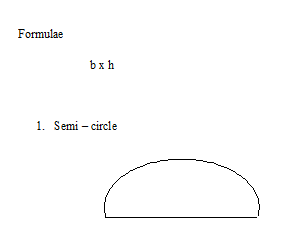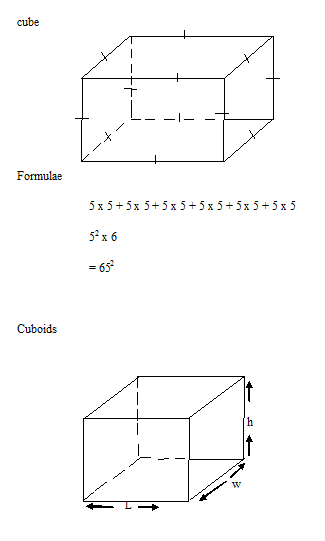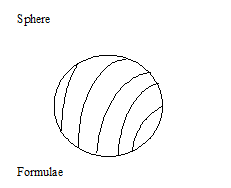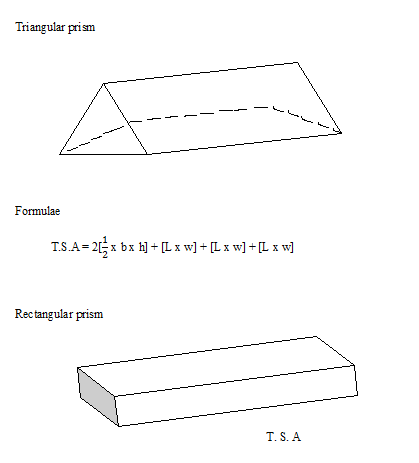# PHY1: AREA

##### This Unit is about the Area as calculated in O level Physics.

AREA

Area is the region under or within a boundary of an object. Area is measured in square units e.g. cm3, m2, mm2.

Where

1km2 =? m2

[1km]2 = [1000m]2

1km2 = 1000m x 1000m

1km2 = 1,000,000m2

And

1m2 =? cm2

[1m] 2 = [100cm]2

1m2 = 1000cm x 100cm

1m2 = 10,000cm2

FORMULAE FOR FINDING AREA OF OBJECTS

ObjectFormulae

1/2  x b x h

RectangleFormulae

Length x width

SquareFormulae

1/2 x r2

QuadrantFormulae

1/4 x r2

SURFACE AREA

It is the total area around the surface of an object. It is obtained by either adding up the area of the faces on using formulae.

EXAMPLESFormulae

2 x r2   = [r +h]xr2## STEM Elearning

We at FAWE have built this platform to aid learners, trainers and mentors get practical help with content, an interactive platform and tools to power their teaching and learning of STEM subjects, more

#### How to find your voice as a woman in Africa

© FAWE, Powered by: Yaaka DN.